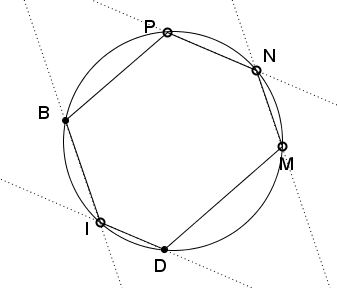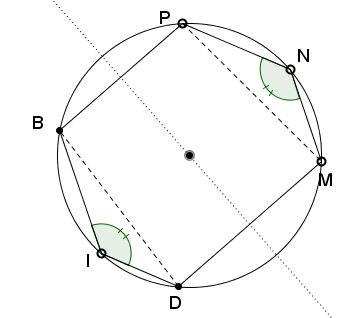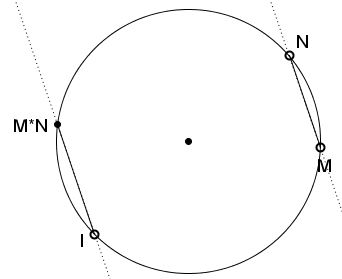# Multiplication of Points on a Circle

### Problem

In a cyclic hexagon $IDMNPB,$ $IB\parallel MN$ and $ID\parallel NP.$Prove that $BP\parallel DM.$

### Proof

$\angle BID=\angle MNP,$ since their sides are parallel. The two angles then are subtended by equal chords: $BD=MN.$If $P$ is the point of intersection of $BD$ and $MN$ (which might be at infinity) and $O$ is the center of the circumscribed circle, then $BD$ and $MN$ are symmetric reflections of each other in $OP.$ This implies that $OP\perp BP$ and also $OP\perp DM,$ implying that $BP\parallel DM.$

### An application

Given circle $(O)$ and point $I\in (O).$ For any two points $M$ and $N$ on $(O)$ define a third point $M*N$ such that the chord from $I$ to $M*N$ is parallel to $MN.$Prove that "*" is a binary operation that defines an abelian group on $(O).$

Indeed, commutativity of "*" is obvious. $I$ plays the role of unity. The inverse of a point is the point symmetric to it in the diameter through $I.$ (This is of course if a tangent to a circle is thought of as passing twice through the point of tangency.)

The statement above helps prove the associativity of "*".

Let there be three points $M,$ $N,$ $P.$ By the definition, the chords from $I$ to $M*N$ and $N*P$ are parallel to $MN$ and $NP,$ respectively.In the cyclic hexagon formed by six points $I,$ $N*P,$ $M,$ $N,$ $P,$ $M*N$ two pairs of opposite sides are parallel. By the statement proved above this implies that the third pair of opposite sides are also parallel. Thus the chords from $I$ parallel to $M*N,P$ and $M,N*P$ coincide, meaning that $(M*N)*P=M*(N*P).$

### Generalization

Telv Cohl has commented on the above statement that it is a particular case of Pascal's theorem, which stipulates that the intersections of the opposite sides of a hexagon inscribed in a conic are collinear. This, in particular, implies that, if two of the intersections lie at infinity, then so is the third. As a consequence, the statement holds for an ellipse, or any other conic.

### Acknowledgment

The problem stemmed from the one posted at the CutTheKnotMath facebook page by Leo Giugiuc (Romania). Leo, posted the problem for an ellipse and supplied an elegant algebraic solution.

### What Can Be Multiplied?